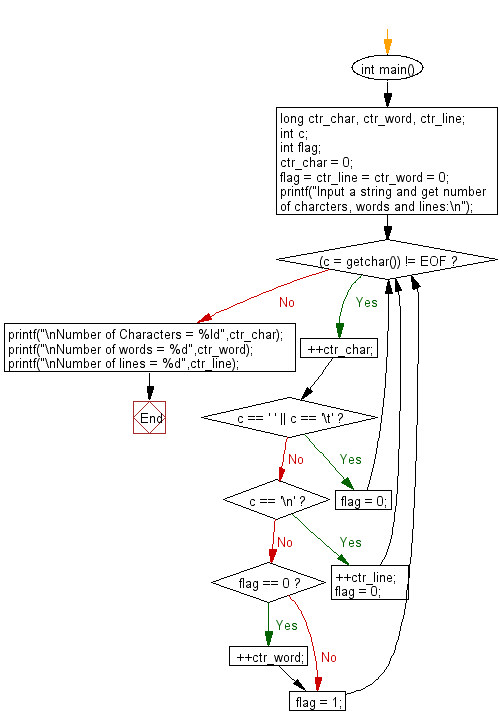﻿ C : Count the number of characters, words and lines in a string

# C Exercises: Count the number of characters, words and lines in a string.

## C Basic Declarations and Expressions: Exercise-97 with Solution

Write a C program that accepts a string and counts the number of characters, words and lines.

Sample Solution:

C Code:

``````#include <stdio.h>

int main() {
long ctr_char, ctr_word, ctr_line; // Variables to count characters, words, and lines
int c; // Variable to hold input characters
int flag; // Flag to track word boundaries

ctr_char = 0; // Initialize the count of characters
flag = ctr_line = ctr_word = 0; // Initialize flag and counts for words and lines

printf("Input a string and get number of characters, words and lines:\n");

// Loop to read characters until end-of-file (EOF) is encountered
while ((c = getchar()) != EOF) {
++ctr_char; // Increment the count of characters

if (c == ' ' || c == '\t') {
flag = 0; // Reset the flag when a space or tab is encountered
} else if (c == '\n') {
++ctr_line; // Increment the count of lines
flag = 0; // Reset the flag on a newline
} else {
if (flag == 0) {
++ctr_word; // Increment the count of words when a new word begins
}
flag = 1; // Set the flag to indicate a word is in progress
}
}

// Print the counts of characters, words, and lines
printf("\nNumber of Characters = %ld", ctr_char);
printf("\nNumber of words = %d", ctr_word);
printf("\nNumber of lines = %d", ctr_line);
}
``````

Sample Output:

```Input a string and get number of characters, words and lines:
The quick brown fox jumps over the lazy dog
^Z

Number of Characters = 44
Number of words = 9
Number of lines = 1
```

Flowchart:C programming Code Editor:

What is the difficulty level of this exercise?

Test your Programming skills with w3resource's quiz.

﻿